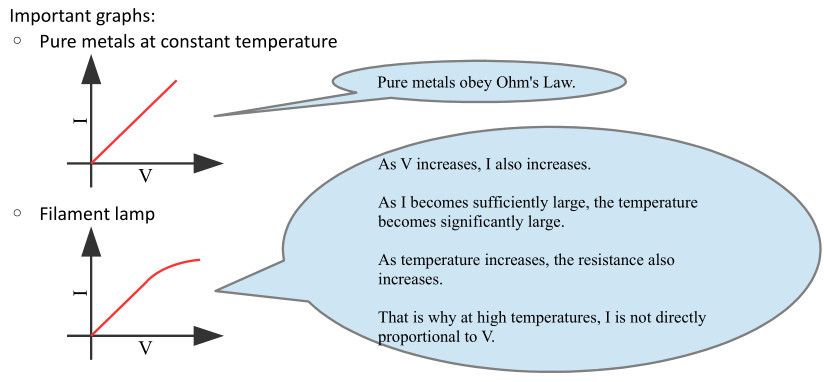Home » Handbook of O Level Physics » UNIT 18: CURRENT ELECTRICITY

# UNIT 18: CURRENT ELECTRICITY

• Current: It is the rate of flow of electric charge.

I = Q / t

• Unit: A

• Current is measured using an ammeter or galvanometer.

• A short-circuit happens when load (resistance) is bypassed and excess current flows due to lack of resistance.

• A short-circuit may cause heating and start a fire.

• Electromotive force (e.m.f.) of a source: It is the energy converted by a source from non-electrical form to electrical form in driving a unit charge round a complete circuit.

e.m.f. = E / Q

• Potential difference between two points: It is the energy converted from electrical to other forms when a unit charge passes between the two points.

V = E / Q

• A comparison between e.m.f. and potential difference:

• Similarities

• Both involve energy and charge

• Both are measured in volts.

• Both are measured by voltmeter.

• Differences

• In e.m.f. the energy change is from non-electrical to electrical. In p.d. the energy change is from electrical to non-electrical.

• e.m.f. is property of source. p.d. is property of part of circuit.

• Volt: The potential difference between two points in a conductor is one volt if one joule of energy is converted from electrical to other forms when one coulomb of positive charge flows through it.

• A voltmeter is connected in parallel.

• Voltmeters have a high resistance.

• An ammeter is connected in series.

• Ammeters have a low resistance.

• Resistance: It is the ratio of potential difference across a material to the current flowing in it.

R = V / I

• Unit: Ω

• Resistors are used in circuits to limit the flow of current. Their types are:

• Fixed resistors

• Variable resistors (rheostats)

• Ohm’s Law: It states that current is directly proportional to potential difference provided that temperature does not change.

V ∝ I

• Limitations: Ohm’s Law is applicable to

• Pure metals

• at constant temperature• Resistance and resistivity

• The constant called resistivity is denoted by ρ.
• Every material has its own value of resistivity.
• Resistivity can be used to tell good conductors from bad.
• It is better to say that copper has low resistivity than to say copper has low resistance.